#MATLAB模糊逻辑(1)

0
0
01. 云栖社区>
2. 博客>
3. 正文

# 第6章  模糊逻辑

## 6.1  隶属函数

### 6.1.1  高斯隶属函数

>>x=0:0.1:10;

>>y=gaussmf(x,[2 5]);

>>plot(x,y)

>>xlabel('gaussmf, P=[2 5]')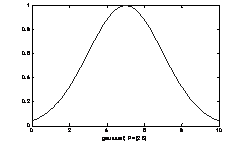6-1

### 6.1.2  两边型高斯隶属函数

6-2

>>x = (0:0.1:10)';

>>y1 = gauss2mf(x, [2 4 1 8]);

>>y2 = gauss2mf(x, [2 5 1 7]);

>>y3 = gauss2mf(x, [2 6 1 6]);

>>y4 = gauss2mf(x, [2 7 1 5]);

>>y5 = gauss2mf(x, [2 8 1 4]);

>>plot(x, [y1 y2 y3 y4 y5]);

>>set(gcf, 'name', 'gauss2mf', 'numbertitle', 'off');

### 6.1.3  建立一般钟型隶属函数

6-3

>>x=0:0.1:10;

>>y=gbellmf(x,[2 4 6]);

>>plot(x,y)

>>xlabel('gbellmf, P=[2 4 6]')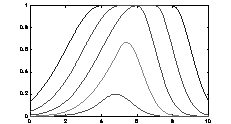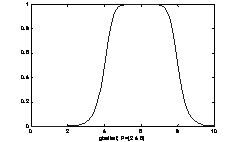图6-2                                                        6-3

### 6.1.4  两个sigmoid型隶属函数之差组成的隶属函数

x是变量，a,c是参数。dsigmf使用四个参数a1，c1，a2，c2，并且是两个sigmoid型函数之差：，参数按顺序列出。

6-4

>>x=0:0.1:10;

>>y=dsigmf(x,[5 2 5 7]);

>>plot(x,y)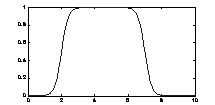图6-4

### 6.1.5  通用隶属函数计算

6-5

>>x=0:0.1:10;

>>mfparams = [2 4 6];

>>mftype = 'gbellmf';

>>y=evalmf(x,mfparams,mftype);

>>plot(x,y)

>>xlabel('gbellmf, P=[2 4 6]')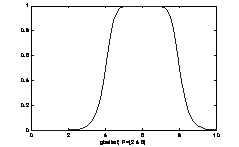图6-5

### 6.1.6  建立П型隶属函数

6-6

>>x=0:0.1:10;

>>y=pimf(x,[1 4 5 10]);

>>plot(x,y)

>>xlabel('pimf, P=[1 4 5 10]')

### 6.1.7  通过两个sigmoid型隶属函数的乘积构造隶属函数

x是变量，a,c是参数。psigmf使用四个参数a1，c1，a2，c2，并且是两个sigmoid型函数之积：，参数按顺序列出。

6-7

>>x=0:0.1:10;

>>y=psigmf(x,[2 3 -5 8]);

>>plot(x,y)

>>xlabel('psigmf, P=[2 3 -5 8]')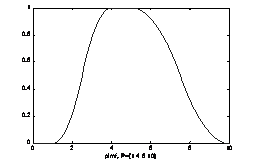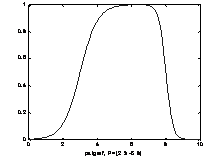图6-6                                                    6-7

### 6.1.8  建立Sigmoid型隶属函数

6-8

>>x=0:0.1:10;

>>y=sigmf(x,[2 4]);

>>plot(x,y)

>>xlabel('sigmf, P=[2 4]')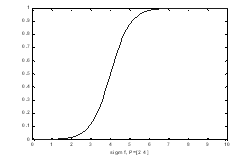6-8

6-9

>>x = (0:0.2:10)’;

>>y1 = sigmf(x,[-1 5]);

>>y2 = sigmf(x,[-3 5]);

>>y3 = sigmf(x,[4 5]);

>>y4 = sigmf(x,[8 5]);

>>subplot(2,1,1),plot(x,[y1  y2  y3  y4]);

>>y1 = sigmf(x,[5 2]);

>>y2 = sigmf(x,[5 4]);

>>y3 = sigmf(x,[5 6]);

>>y4 = sigmf(x,[5 8]);

>>subplot(2,1,2),plot(x,[y1  y2  y3  y4]);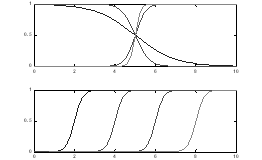6-9

### 6.1.9  建立S型隶属函数

>>x=0:0.1:10;

>>y=smf(x,[1 8]);

>>plot(x,y)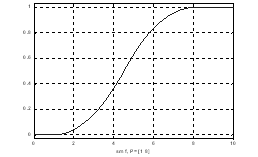6-10

6-11

>>x = 0:0.1:10;

>>subplot(3,1,1);plot(x,smf(x,[2 8]));

>>subplot(3,1,2);plot(x,smf(x,[4 6]));

>>subplot(3,1,3);plot(x,smf(x,[6 4]));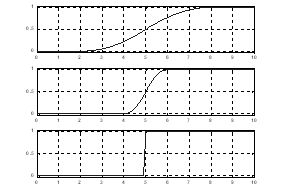6-11

### 6.1.10  建立梯形隶属函数

6-12

>>x=0:0.1:10;

>>y=trapmf(x,[1 5 7 8]);

>>plot(x,y)

>>xlabel('trapmf, P=[1 5 7 8]')

6-13

>>x = (0:0.1:10)’;

>>y1 = trapmf(x,[2 3 7 9]);

>>y2 = trapmf(x,[3 4 6 8]);

>>y3 = trapmf(x,[4 5 5 7]);

>>y4 = trapmf(x,[5 6 4 6]);

>>plot(x,[y1  y2  y3  y4]);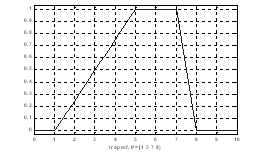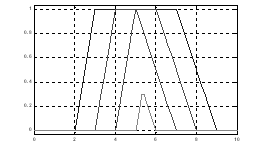图6-12                                                        6-13

### 6.1.11  建立三角形隶属函数

y = trimf(x,[a b c])

6-14

>>x=0:0.1:10;

>>y=trimf(x,[3 6 8]);

>>plot(x,y)

>>xlabel('trimf, P=[3 6 8]')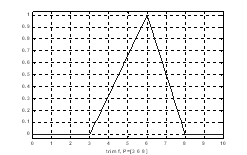6-14

6-15

>>x = (0:0.2:10)’;

>>y1 = trimf(x,[3 4 5]);

>>y2 = trimf(x,[2 4 7 ]);

>>y3 = trimf(x,[1 4 9]);

>>subplot(2,1,1),plot(x,[y1 y2 y3 ]);

>>y1 = trimf(x,[2 3 5]);

>>y2 = trimf(x,[3 4 7]);

>>y3 = trimf(x,[4 5 9]);

>>subplot(2,1,2),plot(x,[y1 y2 y3 ]);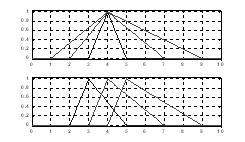6-15

### 6.1.12  建立Z型隶属函数

>>x=0:0.1:10;

>>y=zmf(x,[3 7]);

>>plot(x,y)

>>xlabel('zmf, P=[3 7]')

>>x = 0:0.1:10;

>>subplot(3,1,1);plot(x,zmf(x,[2 8]));

>>subplot(3,1,2);plot(x,zmf(x,[4 6]));

>>subplot(3,1,3);plot(x,zmf(x,[6 4]));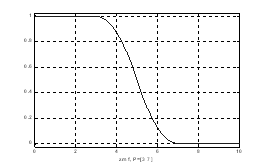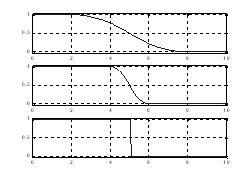图6-16                                                      6-17

### 6.1.13  两个隶属函数之间转换参数

6-18

>>x=0:0.1:5;

>>mfp1 = [1 2 3];

>>mfp2 = mf2mf(mfp1,'gbellmf','trimf');

>>plot(x,gbellmf(x,mfp1),x,trimf(x,mfp2))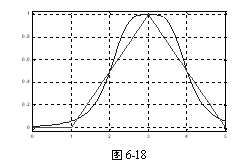### 6.1.14  基本FIS编辑器

fuzzy(fismat)    %使用fuzzy('tipper')，弹出下图FIS编辑器。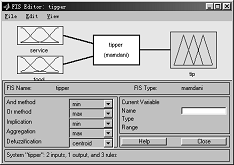·File菜单包括：

New mamdani FIS …   打开新mamdani型系统；

New Sugeno FIS …   打开新Sugeno型系统；

Open from disk …   从磁盘上打开指定的.fis文件系统；

Save to disk    保存当前系统到磁盘上的一个.fis文件上；

Save to disk as …   重命名方式保存当前系统到磁盘上；

Open from workspace …   从工作空间中指定的FIS结构变量装入一个系统；

Save to workspace …   保存系统到工作空间中当前命名的FIS结构变量中；

Save to workspace as …   保存系统到工作空间中指定的FIS结构变量中；

Close windows   关闭GUI

·Edit菜单包括：

Remove variable    删除一个所选的变量；

Undo   恢复当前最近的改变；

·View 菜单包括：

Edit MFs …   调用隶属度函数编辑器；

Edit rules …   调用规则编辑器；

Edit anfis …   只对单输出Sugeno型系统调用编辑器；

View rules …   调用规则观察器；

View surface …   调用曲面观察器。

·And method：为一个定制操作选择min、prod或Custom；

·Or method：为一个定制操作选择max、probor（概率）或Custom；

·Implication method：为一个定制操作选择min、prod或Custom；此项对Sugeno型模糊系统不可用。

·Aggregation method：为一个定制操作选择max、sum、probor或Custom。此项对Sugeno型模糊系统不可用。

·Defuzzification method：对Mamdani型推理，为一个定制操作选择centroid（面积中心法）、bisector（面积平分法）、mom（平均最大隶属度法）、som（最大隶属度最小值法）、lom（最大隶属度最大值法）或Custom。对Sugeno型推理，在wtaver（加权平均）或wtsum（加权和）之间选择。

### 6.1.15  隶属函数编辑器

mfedit(a)

mfedit

mfedit(a)对于FIS结构操作一个MATLAB工作空间变量a。Mfedit可单独弹出没有装入FIS的隶属函数编辑器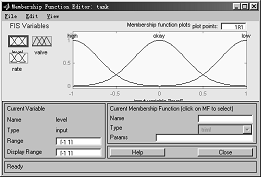·Edit菜单项包括：

Remove current MF   删除当前的隶属度函数；

Remove all MFS   删除当前语言变量的所有隶属度函数；

Undo   恢复当前最近的改变。

·View菜单项包括：

Edit FIS properties…   调用FIS编辑器；

Edit rules…   调用规则编辑器；

View rules…   调用规则观察器；

View surface…   调用曲面观察器。

+ 关注